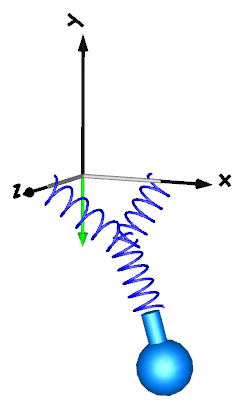# Spring-mass system

This example mirrors that of the modelica three-springs and demonstrates that we can connect mass-less force elements together.The example connects three springs together in a single point. The springs are all massless and do normally not have any state variables, but we can insist on one of the springs being stateful, in this case, we must tell the lower spring component to act as root by setting fixedRotationAtFrame_a = fixedRotationAtFrame_b = true.

using Multibody
using ModelingToolkit
using Plots
using SymbolicIR
using OrdinaryDiffEq

t = Multibody.t
D = Differential(t)
world = Multibody.world

systems = @named begin
body1 = Body(m = 0.8, I_11 = 0.1, I_22 = 0.1, I_33 = 0.1, r_0 = [0.5, -0.3, 0],
r_cm = [0, -0.2, 0], isroot = false)
bar1 = FixedTranslation(r = [0.3, 0, 0])
bar2 = FixedTranslation(r = [0, 0, 0.3])
spring1 = Multibody.Spring(c = 20, m = 0, s_unstretched = 0.1,
r_rel_0 = [-0.2, -0.2, 0.2])
spring2 = Multibody.Spring(c = 40, m = 0, s_unstretched = 0.1,
fixedRotationAtFrame_a = true, fixedRotationAtFrame_b = true)
spring3 = Multibody.Spring(c = 20, m = 0, s_unstretched = 0.1)
end
eqs = [connect(world.frame_b, bar1.frame_a)
connect(world.frame_b, bar2.frame_a)
connect(bar1.frame_b, spring1.frame_a)
connect(bar2.frame_b, spring3.frame_a)
connect(spring2.frame_b, body1.frame_a)
connect(spring3.frame_b, spring1.frame_b)
connect(spring2.frame_a, spring1.frame_b)]

@named model = ODESystem(eqs, t, systems = [world; systems])
ssys = structural_simplify(IRSystem(model))
prob = ODEProblem(ssys, [], (0, 10))

sol = solve(prob, Rodas4(), u0=prob.u0 .+ 1e-1*randn(length(prob.u0)))
@assert SciMLBase.successful_retcode(sol)

plot(sol, idxs = [body1.r_0...])